# IIT JAM Chemistry - MCQ Test 5

## 60 Questions MCQ Test MOCK Test Series for IIT JAM Chemistry | IIT JAM Chemistry - MCQ Test 5

Description
This mock test of IIT JAM Chemistry - MCQ Test 5 for Chemistry helps you for every Chemistry entrance exam. This contains 60 Multiple Choice Questions for Chemistry IIT JAM Chemistry - MCQ Test 5 (mcq) to study with solutions a complete question bank. The solved questions answers in this IIT JAM Chemistry - MCQ Test 5 quiz give you a good mix of easy questions and tough questions. Chemistry students definitely take this IIT JAM Chemistry - MCQ Test 5 exercise for a better result in the exam. You can find other IIT JAM Chemistry - MCQ Test 5 extra questions, long questions & short questions for Chemistry on EduRev as well by searching above.
QUESTION: 1

Solution:
QUESTION: 2

Solution:
QUESTION: 3

### For the reaction the value of rate of disappearance of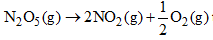is given as 6.25 × 10–3 mol L–1S–1. The rate of formation of NO2 and O2 is given respectively as:

Solution:
QUESTION: 4

In the synthesis of ammonia by Haber process, if 60 moles of ammonia is obtained in one hour, then the rate of disappearance of Nitrogen is:

Solution:
QUESTION: 5

For a first order reaction A → P, the temperature (T) dependent rate constant (k) was found to follow the equation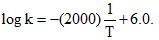The pre-exponential factor A and the activation energy Ea, respectively, are:

Solution:
QUESTION: 6

In the case of alkali metals, the covalent character decreases in the order:

Solution:
QUESTION: 7

Which of the following does not illustrate the anomalous properties of lithium

Solution:
QUESTION: 8

On heating anhydrous Na2CO3.........is evolved:

Solution:

The chemical formula of sodium carbonate (washing soda) is Na2​CO3​. When it is heated it undergoes a thermal decomposition reaction. This takes place above 500 degree C. The reaction of decomposition is Na2​CO3​(s)→Na2​O(s)+CO2​(g)

The name of the first reaction product Na2​O is Sodium Oxide which is solid and used in glass making and the name of the second reaction product is Carbon Dioxide which is a gas.

QUESTION: 9

Which of the following sulphates have the highest solubility in water:

Solution:
QUESTION: 10

The element that does not show catenation among the following p-block elements is:

Solution:
QUESTION: 11

A quantity of 0.50 mole of an ideal gas at 20°C expands isothermally against a constant pressure of  2.0 atm from 1.0 L to 5.0 L. Entropy change of the system is:

Solution:
QUESTION: 12

Given the molecular formula of the hexa-coordinated complexes (A) CoCl3.6NH3 (B) CoCl3.5NH3   (C) CoCl3.4NH3. If the number of coordinated NH3 molecules in A, B and C respectively are 6, 5 and 4 the primary valency in (A), (B) and (C) are:

Solution: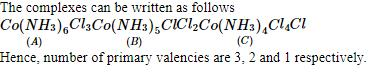QUESTION: 13

Ketones react with Mg-Hg over water gives

Solution:
QUESTION: 14

Which one of the following statement for order of reaction is not correct:

Solution:
QUESTION: 15

t1/4 can be taken as the time taken for the concentration of a reactant to drop to 3/4 of its initial value. If the rate constant for a first order reaction is K, the t1/4 can be written as:

Solution:
QUESTION: 16

The compound that undergoes decarboxylation most readily under mild condition is:

Solution:
QUESTION: 17

Consider the acidity of the carboxylic acids:

(I) PhCOOH
(II) o-NO2C6H4COOH
(III) p-NO2C6H4COOH
(IV) m-NO2C6H4COOH

Which of the following order is correct:

Solution:
QUESTION: 18

Starting with one mole of a compound A it is found that the reaction is 3/4th complete in one hour. If the reaction is first order, the rate constant is:

Solution:
QUESTION: 19

Find the organic acid produced from the below reaction: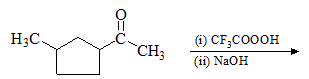Solution:
QUESTION: 20

The diketone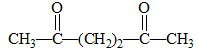on intramolecular aldol condensation gives the final product.

Solution:
QUESTION: 21

Nitrogen forms how many oxide?

Solution:
QUESTION: 22

Which of the following does not have optical isomer

Solution:
QUESTION: 23

Which one is not correct:

Solution:
QUESTION: 24

Which of the following complex ions is expected to absorb visible light:

Solution:
QUESTION: 25

The reaction [Fe(CNS)6]3– → [FeF6]3– takes place with

Solution:
QUESTION: 26

The crystal field stabilization energy (CFSE) is the highest for:

Solution:

Higher the oxidation state of the metal, greater the crystal field splitting energy . In options (a), (b) and (d), Co is present in +2+2 oxidation state and in (c ) it is present in +3+3 oxidation state and hence has a higher value of CFSE.

QUESTION: 27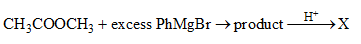The product X is

Solution:
QUESTION: 28

An optically active compound having molecular formula C8H16 on ozonolysis gives acetone as one of       the products. The structure of the compound is:

Solution:
QUESTION: 29

The major organic product formed from the following reaction: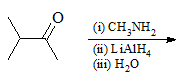Solution:
QUESTION: 30

For the redox reaction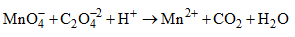The correct coefficients of the reaction for the balanced reaction are: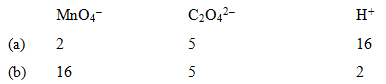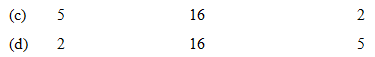Solution:
*Multiple options can be correct
QUESTION: 31

For a first order reaction:

Solution:
*Multiple options can be correct
QUESTION: 32

The following statement(s) is(are) correct:

Solution:
*Multiple options can be correct
QUESTION: 33

For the first order reaction

2N2O5(g) → 4NO2 (g) + O2 (g)

Solution:
*Multiple options can be correct
QUESTION: 34

The rate of law for the reaction

RCI + NaOH → ROH + NaCl

is given by rate = k[RCl]. The rate of this reaction

Solution:
*Multiple options can be correct
QUESTION: 35

For the reaction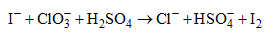The correct statement(s) in the balanced equation is/are:

Solution:
*Multiple options can be correct
QUESTION: 36

Reduction of the metal centre in aqueous permangnate ion involves:

Solution: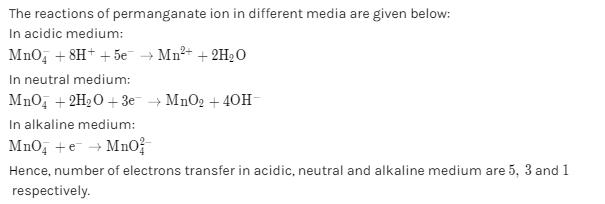*Multiple options can be correct
QUESTION: 37

In the reaction of sodium thiosulphate with I2 in aqueous medium the equivalent weight of sodium thiosulphate is equal to:

Solution:
*Multiple options can be correct
QUESTION: 38

In which of the following arrangements the sequence is not strictly according to the property written against it:

Solution:
*Multiple options can be correct
QUESTION: 39

Which one of the following is wrongly matched:

Solution:
*Multiple options can be correct
QUESTION: 40

Red precipitate is obtained when ethanol solution of dimethylglyoxime is added to ammoniacal   Ni(II). Which of the following statement is/are true: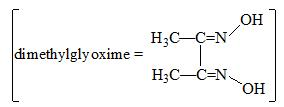Solution:
*Answer can only contain numeric values
QUESTION: 41

If the activation energy for the forward reaction is 150 kJ mol–1 and that of the reverse reaction is 260 kJ mol–1, what is the enthalpy change (kJ mol–1) for the reaction:

Solution:
*Answer can only contain numeric values
QUESTION: 42

The rate of a reaction is doubled for every 10°C rise in temperature. The increase in reaction rate as a result of temperature rise from 10°C to 100°C is:

Solution:
*Answer can only contain numeric values
QUESTION: 43

Cyclopropene rearranges to form propene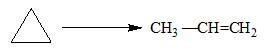This follows first order kinetics. The rate constant is 2.714 × 10–3 sec–1. The initial concentration   of cyclopropane is 0.29 M. What will be the concentration (M) of cyclcopropene after 100 sec:

Solution:
QUESTION: 44

A gaseous hypothetical chemical equation 2A → 4B +C is carried out in a closed vessel. The concentration of B is found to increase by 5 × 10–3 mol l–3 in 10 second. The rate of appearance of B is(mol l–3 sec–1):

Solution:
QUESTION: 45

1 mole of an ideal gas for which CV = 3/2 R is heated reversibly at a constant pressure of 1 atm from  25°C to 100°C. The ΔH(cal) is:

Solution: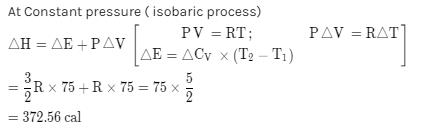*Answer can only contain numeric values
QUESTION: 46

The number of isomers possible for distributed borazine, B3N3H4X2 is:

Solution:
*Answer can only contain numeric values
QUESTION: 47

The possible number of optical isomers in [Co(en)2Cl2]+ are:

Solution:
*Answer can only contain numeric values
QUESTION: 48

The magnetic moment of a transition metal ion is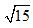BM. Therefore the number of unpaired electrons present in it is:

Solution:
*Answer can only contain numeric values
QUESTION: 49

The spin only magnetic moment value (in Bohr magneton units) of Cr(CO)6 is:

Solution:
*Answer can only contain numeric values
QUESTION: 50

The total number of possible isomers for the complex compound [CuII(NH3)4][PtIICl4] are:

Solution: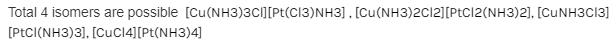*Answer can only contain numeric values
QUESTION: 51

The decomposition of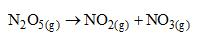Proceeds as a first order reaction with a half-life period of 30 seconds at a certain temperature. If the       initial concentration [N2O5] = 0.4 M, what is the rate constant of the reaction(sec-1):

Solution:
*Answer can only contain numeric values
QUESTION: 52

If a substance with half life 3 days is taken at other place in 12 days. What amount of substance   is left now:

Solution:
*Answer can only contain numeric values
QUESTION: 53

The rate of reaction between two reactants A and B decreases by a factor of 4 if the concentration of reactant B is doubled. The order of this reaction with respect to reactant B is:

Solution:
QUESTION: 54

The rate of disappearance of SO2in the reaction 2SO2 + O→ 2SOis 1.28 × 10–3 g/sec then the rate of formation (g/sec) of SO3 is:1.28 × 10–3

Solution:
*Answer can only contain numeric values
QUESTION: 55

Number of P-O bonds in P4O10 is:

Solution: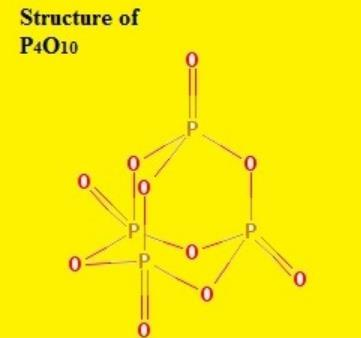From figure it is clear that we have 12 P-O and 4 P=O bonds.

*Answer can only contain numeric values
QUESTION: 56

Heat obtained due to expansion of 1 mole of H2 gas at 1000 K from 10 L to 100 L under isothermal        reversible condition is absorbed by an engine having a sink at 300 K. Useful work (cal) obtained is:

Solution:
*Answer can only contain numeric values
QUESTION: 57

At constant pressure 200 g of water is heated from 10°C to 20°C. Thus, increase in entropy is (molar heat capacity of water (Joules) at constant pressure is 75.3 JK–1 mol–1):

Solution:
*Answer can only contain numeric values
QUESTION: 58

Carnot cycle is said to have 25% efficiency when it operates between T (source) and 300 K (sink), Temperature T is (in K):

Solution:
*Answer can only contain numeric values
QUESTION: 59

The value of log10 K for the reaction A <=> B is (Given, Δt298 K = – 54.07 kJ mol–1,  Δt298 K = 10 JK–1 mol–1, R = 8.314 JK–1 mol–1; 2.303 × 8.314 × 298 = 5705):

Solution: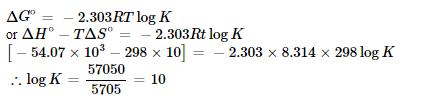*Answer can only contain numeric values
QUESTION: 60

1 mole of an ideal gas is allowed to expand reversibly and adiabatically from a temperature of 27°C.  The work done is 3 kJ. The final temperature (K) of the gas is equal to (CV = 20 J mol–1K–1):

Solution: